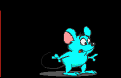## Topics in low dimensional topology and Topological Quantum Field Theories

(part of the MRI Masterclass Quantum groups, affine Lie algebras and their applications)

Tought by Michael Müger and Hessel Posthuma.

### Location and time:

REC-C 2.10, Mondays 14:00 - 17:00

### Prerequisites:

Algebra: Linear algebra, Tensor product of vector spaces. Basics of categories. [Lang]
General Topology: Hausdorff, 2nd countability, compactness, connectedness, classification of surfaces (d=2 topological manifolds) [Mun]
Differential Topology: smooth manifolds and maps, tangent space, boundary, orientation, some Morse theory would be helpful [Hir]

### Subjects treated

10.9.: Introduction, Motivation, Background. Pedestrian definition of a TQFT [At, L, K]
17.9.: Pedestrian definition of a TQFT (conclusion), example: TQFT in 0+1 dimensions
24.9.: The category of cobordisms. General stuff on tensor categories and functors.
1.10.: More category stuff: monoidal natural transformations, (monoidal) equivalence, symmetries and braidings. TQFT as tensor functor, relation to Atiyah's defin. Group cohomology, in particular H^3(G,A), classification of the group categories C(G,α)
8.10.: (Hessel) TQFTs in 1+1 dimensions vs. commutative Frobenius algebras.
15.10.: TQFTs in d=1+1 (conclusion). Simplicial stuff: semisimplicial sets and their (co)homology, group (co)homology, singular (co)homology.
24.10.: Completion of simplicial stuff.
Homework: All exercises in the lecture notes. (Until november 5.)
29.10.: Final comments on simplicial stuff. Pachner moves. Invariants of 2-manifolds via triangulation [La].
Homework (hand in on 12.11.)
5.11.: TQFT in 1+1 dimensions from triangulation [La,FHK]. Graphical notation for (braided) tensor categories.
Homework (hand in on 19.11.): Exercises 7.16, 7.18, 7.19.
12.11.: The commutative Frobenius algebra of a triangulation TQFT (d=1+1). Pacher moves in d>=3.
Homework (hand in on 26.11.): Exercises 6.30, 6.39
19.11.: Final comments on triangulation in d=1+1. More on categories: semisimplicity, duals, sphericity.
Homework (hand in on 26.11.): Exercises 6.25 and 6.51.
26.11.: 3-Manifold invariant from triangulation: Definition and triangulation-independence (overview). Comments on the triangulation TQFT. [BW,GK]
Homework (hand in on 3.12.): The two exersices in Section 7.2 and Exercises A.65 and A.66 (if you haven't done them already).
3.12. (Hessel): TQFTs in all dimensions I [FQ]. LECTURE NOTES
Homework (hand in on 10.12.): All (four) exercises in the notes.
10.12. (Hessel): TQFTs in all dimensions II [FQ]
17.12. (Hessel/Michael): Outlook: TQFT in 2+1 dimensions from surgery (Reshetikhin/Turaev) [T,BK]

## Literature:

Brief lecture notes on singular homology

### Books:

Algebra:
[Lang] S. Lang: Algebra. 3rd ed. Addison Wesley or Springer
General topology:
[Mun] J.R. Munkres: Topology. 2nd ed., Prentice Hall, 2000.
Algebraic topology:
J.P. May: A concise course in algebraic topology. Chicago University Press, 1999.
A. Hatcher: Algebraic topology. Cambridge University Press, 2002.
G.E. Bredon: Topology and geometry. Springer, 1993.
Differential topology:
[Hir] M.W. Hirsch: Differential topology. Springer.

Low dimensional topology and TQFT:
[K]: J. Kock: Frobenius algebras and 2D topological quantum field theories. Cambridge University Press.
[PS] V.V. Prasolov and A.B. Sossinsky: Knots, links, braids and 3-manifolds. American Mathematical Society.
[T] V. Turaev: Quantum invariants of knots and 3-manifolds. Walter de Gruyter.
[BK] B. Bakalov and A. Kirillov Jr.: Lectures on tensor categories and modular functors. American Mathematical Society
[Kas] C. Kassel: Quantum groups. Springer.
[KRT] C. Kassel, M. Rosso and V. Turaev: Quantum groups and knot invariants. SMF.
[Li] W.B.R. Lickorish: An introduction to knot theory. Springer.

### Articles:

Two useful reviews by C. Blanchet and V. Turaev: here and here.
[A] M. Atiyah: Topological Quantum Field Theory. Publ. Math. IHES 68, 175-186 (1988).
[BW1] J. W. Barrett and B. W. Westbury: Spherical categories. Adv. Math. 143, 357-375 (1999)
[BW2] J. W. Barrett and B. W. Westbury: Invariants of piecewise-linear 3-manifolds. Trans. Amer. Math. Soc. 348, 3997-4022 (1996)
[FQ] D.S. Freed and F. Quinn: Chern-Simons theory with finite gauge group. Commun. Math. Phys. 156, 435-472 (1993)
[FHK] M. Fukuma, S. Hosono and H. Kawai: Lattice topological field theory in two dimensions. Commun. Math. Phys. 161, 157-175 (1994)
[GK] S. Gelfand and D. Kazhdan: Invariants of three-dimensional manifolds. Geom. Funct. Anal. 6, 268-300 (1996).
[La] R. Lawrence: An introduction to Topological Field Theory. Proc. Symp. Pure Math 51, 89-128 (1996).
[Q] F. Quinn: Lectures on axiomatic topological quantum field theory. In: Daniel S. Freed and Karen K. Uhlenbeck (eds.): Geometry and quantum field theory. IAS/Park City Mathematics Series, 1. American Mathematical Society, Providence, RI, 1995.